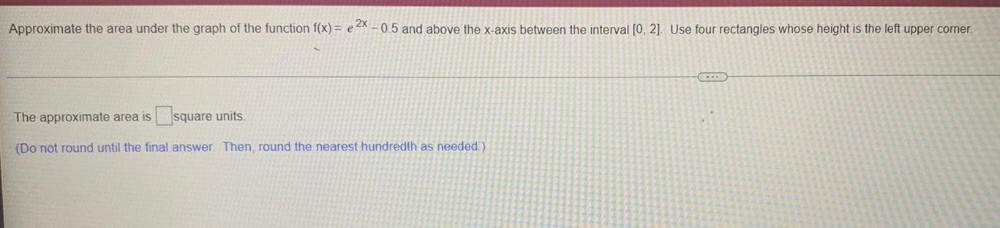Question:

# Approximate the area under the graph of the function f(x) = e 2% - 0.5 and above the x-axis between the interval [0, 2]. Use fouApproximate the area under the graph of the function f(x) = e 2% - 0.5 and above the x-axis between the interval [0, 2]. Use four rectangles whose height is the left upper corner. The approximate area is square units. (Do not round until the final answer. Then, round the nearest hundredth as needed )